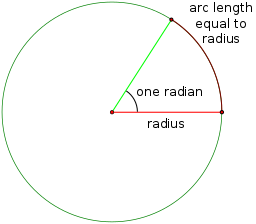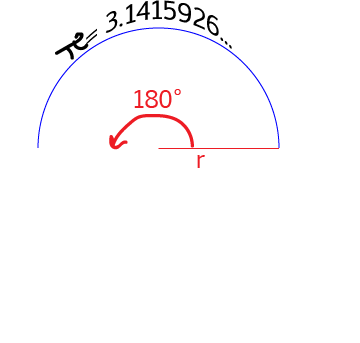# Year 10+ Lines and Angles

### ChaptersAs you start to do more complicated mathematics, you will be introduced to the radian as a unit for measuring angles. A radian is the angle formed at the centre of a circle by an arc with the same length as the radius of the circle. Mathematicians like radians for a number of reasons:

1. It is a measurement based on the radius of the circle. Degrees really have nothing to do with the circle.
2. As $x$ gets close to zero, $\sin (x)$ and $\tan (x)$ get close to $x$.
3. Calculus works much more nicely with radians than with degrees. We don't have to multiply by pesky constants.

## What Does One Radian Look Like?

So that you can see what one radian looks like, here's an angle that measures 1 radian. 1 radian works out to be $57.2958\dots^\circ$.### Why Such a Funny Number?

Remember that one radian is the angle at the centre of a circle formed by an arc that has the same length as the radius of a circle? Well, the circumference of a circle is given by $2\pi$ times its radius. So, a full revolution is equal to $2\pi \text{ radians}$. In other words, $360^\circ = 2 \pi \text{ radians}$, and so $1 \text{ radian} = \dfrac{360}{2\pi} = \dfrac{180}{\pi}^\circ = 57.2958\dots^\circ$.

Since there are $2\pi$ radians in a whole circle, there are $\pi \text{ radians}$ in a semicircle. This tells us that $\pi \text{ radians} = 180^\circ$, as shown in the picture below## The Sizes of Some other Common AnglesA full turn (a full revolution) is $360^\circ$. This is also the angle at the centre of a whole circle. So, a full turn is equal to $2\pi \text{ radians}$.A straight angle (a half turn) is $180^\circ$. This is the angle at the centre of a semicircle, so a straight angle is equal to $\pi \text{ radians}$.A right angle (also known as a quarter turn) is $90^\circ$. This is also the angle at the centre of a quarter circle, so a right angle is equal to $\dfrac{\pi}{2} \text{ radians}$.

In general, to convert from radians to degrees we multiply the angle in radians by $180^\circ$ and divide it by $\pi$.

To convert from degrees to radians, we multiply the angle in degrees by $\pi$ and divide it by $180$.

The table below gives a list of some common angles in degrees, and their equivalents in radians.

$0^\circ$ $0$ $0$
$30^\circ$ $\dfrac{\pi}{6}$ $0.524$
$45^\circ$ $\dfrac{\pi}{4}$ $0.785$
$60^\circ$ $\dfrac{\pi}{3}$ $1.047$
$90^\circ$ $\dfrac{\pi}{2}$ $1.571$
$120^\circ$ $\dfrac{2\pi}{3}$ $2.094$
$135^\circ$ $\dfrac{3\pi}{4}$ $2.356$
$150^\circ$ $\dfrac{5\pi}{6}$ $2.618$
$180^\circ$ $\pi$ $3.142$
$270^\circ$ $\dfrac{3\pi}{2}$ $4.712$
$360^\circ$ $2\pi$ $6.283$

## Conclusion

### Description

In this mini book, you will learn about

1. Alternate angles
2. Cointerior angles
4. Vertically Opposite Angles

and several other topics related to lines and angles.

### Audience

Year 10 or higher

### Learning Objectives

To learn the basics of Lines and Angles stream of Geometry

Author: Subject Coach
You must be logged in as Student to ask a Question.